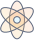# Master Common Statistical Tests with Picmonic for Medicine

## With Picmonic, facts become pictures. We've taken what the science shows - image mnemonics work - but we've boosted the effectiveness by building and associating memorable characters, interesting audio stories, and built-in quizzing.

### Common Statistical Teststantheman
6 KEY FACTS
Determine If Comparing Numerical Or Categorical Variables
Scattergories and Abacus on opposite ends of room

One of the first steps in selecting the appropriate statistical analysis method is determining if the variables to be compared are numerical or categorical. For example, if a study were to be done on blood pressure and patients were defined as having either high or low blood pressures, they are being evaluated according to categorical variables. If instead patients were defined by specific blood pressure ranges (systolic BP 100-109, 110-119, 120-129 etc), they would be evaluated according to numerical variables.

NUMERICAL VARIABLES
T-Test For Comparing Means Of Two Groups
Two children drinking tutu-tea

A t-test is ideal for comparing mean values (or averages) between two groups to determine if there is a significant difference between the two groups. One example of this would be to compare the numerical mean systolic blood pressure in men versus women. Importantly, the t-test can only be applied when the test statistic follows a normal bell-curve distribution.

ANOVA For Comparing Means In Greater Than Three Groups
Three children in three-tree watching supernova

ANOVA, which is short for analysis of variance, is a stastical model used to analyze the numerical differences among greater than three groups in a study. One application of this would be comparing the mean blood pressure between members of three or more ethnic groups.

Comparing Mean Values On Normal/Bell Distribution
Mean-kid ringing bell

When selecting a statistical test to compare numerical variables, it is important to remember that these tests are only useful when comparing mean values between groups, in which the numerical outcomes result in a normal bell-curve distribution.

CATEGORICAL VARIABLES
Chi-Squared Test For Comparing Categorical Outcomes In Large Sample Sizes
Large child under Chi-city square flag

The chi-squared test is used to determine if there is a statistically significant difference between frequencies or proportions of outcomes in one or more categories. For example, the chi-squared test would be appropriate to use to compare what percentage of multiple ethnic groups have high blood pressure. It is important to note that although percentage and proportion are numerical values, the variable actually being studied, in this example blood pressure, is defined as high or not high and is therefore categorical.

Fisher's Exact Test For Comparing Categorical Outcomes In Small Sample Sizes
Small child-fisher

Fisher's exact test is a statistical test used to analyze differences in percentages of categorical outcomes between groups and is specifically used for small sample sizes, as opposed to the chi-squared test which is used to analyze differences in categorical outcomes between large groups. One example of this would be comparing the percentage of individuals with hypertension in a group of 20 women versus a group of 20 men.

Unlock all 6 facts & definitions with Picmonic Free!

## Take the Common Statistical Tests Quiz

Picmonic's rapid review multiple-choice quiz allows you to assess your knowledge.

## Picmonic for Medicine CoversMedicine (MD/DO)
1,800+ Videos
21,000+ Facts

## Our Story Mnemonics Increase Mastery and Retention

Memorize facts with phonetic mnemonics

Unforgettable characters with concise but impactful videos (2-4 min each)

### Ace Your Medicine (MD/DO) Classes & Exams with Picmonic:

#### Over 1,240,000 students use Picmonic’s picture mnemonics to improve knowledge, retention, and exam performance.